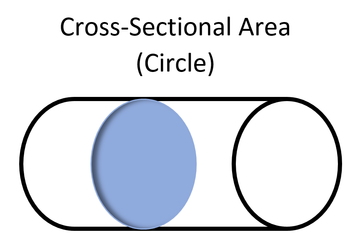# Cross-sectional areaFigure 1. The cross-sectional area of a cylinder is equal to the area of a circle if cut parallel to the circular base.

The cross-sectional area is the area of a two-dimensional shape that is obtained when a three-dimensional object - such as a cylinder - is sliced perpendicular to some specified axis at a point.

For example, the cross-section of a cylinder - when sliced parallel to its base - is a circle. Thus, the cross-sectional area of this slice is the area of a circle with the radius equal to the radius of the provided cylinder.

## Uses

The cross-sectional area of some shape is important in many different applications. The cross-section of a series of pipes carrying water helps to determine the speed and pressure with which the water flows through the pipe, especially if there is some change in the cross-sectional area of the pipe at some point. Using Bernoulli's equation, changes in the cross-sectional area of a pipe can be used to determine how other variables such as water pressure and speed must change to accommodate for this pipe change. As a result of this, cross-sectional area of pipes must be taken into account when building hydroelectric dams - particularly the penstocks - for hydroelectric power generation.

Additionally, the cross-sectional area is important when looking at nuclear reactions. The cross-section of some nucleus is used to define the effective size of a nucleus, and thus this value can be used to express the probability of some nuclear reaction taking place. As well, the neutron cross section is particularly important as it expresses how likely a reaction between a neutron and a target nucleus is, the basis for nuclear fission.

## Authors and Editors

Kailyn Stenhouse, Jason Donev
Last updated: February 18, 2016
Get Citation